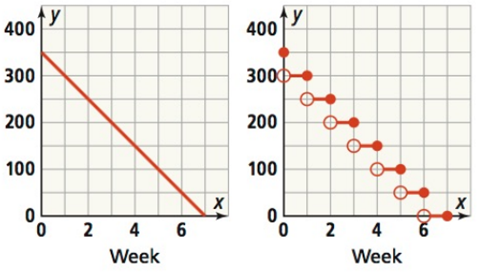Mathematics
Easy

Question

# Mia had \$ 350 in her bank account at the beginning of the school year. Each week she withdraws \$ 50. Two graphs model the situationWrite a function for each graph.

## f(x) = -50x + 350 , 0 ≤ x ≤ 7 ; f(x) = 350 – 50 ceiling(x) , 0 ≤ x ≤ 7f(x) = 50x + 300 , 0 ≤ x ≤ 7 ; f(x) = 300 – 50 ceiling(x) , 0 ≤ x ≤ 7 f(x) = -50x + 300 , 0 ≤ x ≤ 7 ; f(x) = 300 + 50 floor(x) , 0 ≤ x ≤ 7 f(x) = 50x + 350 , 0 ≤ x ≤ 7 ; f(x) = 300 – 50 floor(x) , 0 ≤ x ≤ 7Hint:

## The correct answer is: f(x) = -50x + 350 , 0 ≤ x ≤ 7 ; f(x) = 350 – 50 ceiling(x) , 0 ≤ x ≤ 7

### Related Questions to study#### With Turito Foundation.#### Get an Expert Advice From Turito.